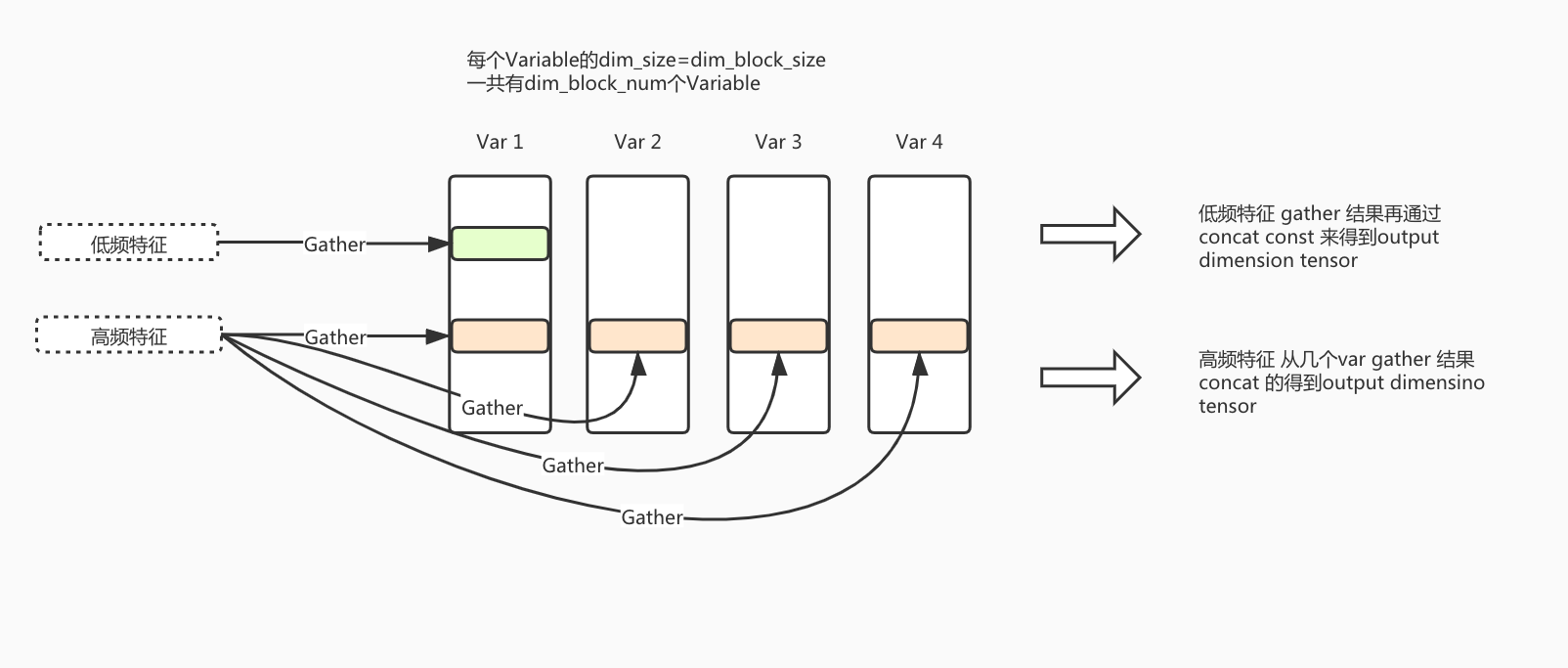# Dynamic-dimension Embedding Variable

## 背景

Dynamic Dimension EmbeddingVariable功能依据特征的频度来给Embedding动态分配维度；高频高维度，低频低维度； 高频特征可以被给到很高的维度而不担心欠拟合问题， 而低频特征因为给定低维度embedding，一定程度上起到了正则的作用，既缓解了过拟合的问题，而且可以极大程度节省内存(低频长尾特征的数量占据绝对优势) ​Dynamic-dimension Embedding Variable示例图

## 用户接口

def get_dynamic_dimension_embedding_variable(
name,
embedding_block_dimension, # 表示单元EV的dimension
embedding_block_num, # 新增，表示单元EV的数目
key_dtype=dtypes.int64,
value_dtype=None,
initializer=None,
regularizer=None,
trainable=True,
collections=None,
caching_device=None,
partitioner=None,
validate_shape=True,
custom_getter=None,
constraint=None,
steps_to_live=None,
steps_to_live_l2reg=None,
l2reg_theta=None
)


def sparse_column_with_dynamice_dimension_embedding(
column_name, dtype=dtypes.string, partition_num=None, steps_to_live=None,
embedding_block_dimension, # 表示单元EV的dimension
embedding_block_num, # 新增，表示单元EV的数目
)


dynamic dimension embedding variable的大部分参数都和get_embedding_variable相同，下面解释dynamic dimension embedding variable中主要的参数：

• embedding_block_dimension: 每一个block的维度

• embedding_block_num: 一个特征的embedding最多可以有多少个block

'''
blocknums: 新加参数，是一个和ids一一对应的tensor，代表每个id需要去几个fae的ev blocks中去找
example:

embedding_block_num=4

ids=[21,34,78,99,56],blocknums=[4, 1, 4, 3, 1]

[[1. 1. 1. 1. 1. 1. 1. 1. 1. 1. 1. 1. 1. 1. 1. 1. 1. 1. 1. 1. 1. 1. 1. 1.]
[1. 1. 1. 1. 1. 1. 0. 0. 0. 0. 0. 0. 0. 0. 0. 0. 0. 0. 0. 0. 0. 0. 0. 0.]
[1. 1. 1. 1. 1. 1. 1. 1. 1. 1. 1. 1. 1. 1. 1. 1. 1. 1. 1. 1. 1. 1. 1. 1.]
[1. 1. 1. 1. 1. 1. 1. 1. 1. 1. 1. 1. 1. 1. 1. 1. 1. 1. 0. 0. 0. 0. 0. 0.]
[1. 1. 1. 1. 1. 1. 0. 0. 0. 0. 0. 0. 0. 0. 0. 0. 0. 0. 0. 0. 0. 0. 0. 0.]]
'''
def embedding_lookup(
params,
ids,
partition_strategy="mod",
name=None,
blocknums=None
)


## 使用示例

### 动态维度调整策略

$freq=\frac{\beta*freq_acc + (1.0-\beta)*freq_current_speed}{(1.0-\beta^t)}$ ， t是freq_acc被reset的次数

1. 对所有freq_acc超过一个阈值的特征进行ranking（该阈值可以设置为0.000001），超过阈值的特征的数量记为total_rank。

2. 考虑到推荐场景中的特征频次分布符合幂律分布，我们给出一个指数分布来划分阈值空间，即给出一个p，得到一个$[p^0,p^1,....,p^{n+1}]$，其中$1<p$，n是blocknum的数量。

3. 对于每一个$k\in[1,....,n+1]$，计算$rank =(p^{n+1-k}-p^0)/(p^{n+1}-p^0) * total_rank$，然后根据freq_acc选出top-rank个freq_acc, 这之中的最小值即是第i个block对应的阈值。

4. 对于每一个特征，将freq与阈值比较即可决定每个对应的embedding的长度。